# SQL Query to Remove Decimal Values

• Last Updated : 23 Sep, 2021

Decimal values are those values that have “float” as a datatype.

There are various methods to remove decimal values in SQL:

Attention reader! Don’t stop learning now. Learn SQL for interviews using SQL Course  by GeeksforGeeks.

• Using ROUND() function: This function in SQL Server is used to round off a specified number to a specified decimal places
• Using FLOOR() function: It returns the largest integer value that is less than or equal to a number.
• Using CAST() function: The explicit conversion has to be done in SQL Server using Cast or Convert function.

STEP 1: Creating a database

Use the below SQL statement to create a database called geeks:

Query:

`CREATE DATABASE geeks;`

Step 2:  Using the database

Use the below SQL statement to switch the database context to geeks:

Query:

`USE geeks;`

Step 3: Table definition

We have the following geeks for geeks table in our geek’s database.

Query:

```CREATE TABLE geeksforgeeks(
NAME VARCHAR(10),
MARKS float);```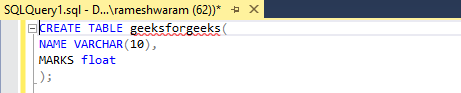Step 4: Insert data into a table

Query:

`INSERT INTO geeksforgeeks VALUES ('ROMY',80.9),('MEENAKSHI',86.89),('SHALINI',85.9),('SAMBHAVI', 89.45);`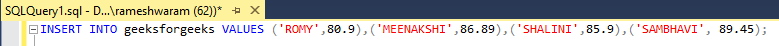Step 5:Check value of the table

Content of the table can be viewed using the SELECT command.

Query:

`SELECT * FROM geeksforgeeks;`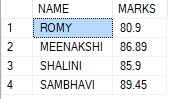Step 6:Use function to remove decimal values

### By using Round() function

• ROUND(): This function rounds a number to the specified decimal places. If we want to remove all the decimal values, we will round it to decimal place 0.

Syntax:

`ROUND(Value, decimal_place)`

Query:

`SELECT NAME, ROUND(MARKS,0) AS MARKS FROM geeksforgeeks;`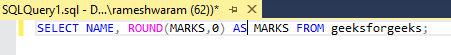Output: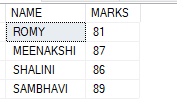80.0 is rounded to 81 as 81 is the nearest integer value.

### By using the FLOOR() function

• FLOOR(): This function returns the largest integer value which is less than or equal to the value used as a parameter.

Syntax:

` FLOOR(value)`

Query:

`SELECT NAME, FLOOR(MARKS) AS MARKS FROM geeksforgeeks;`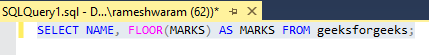Output: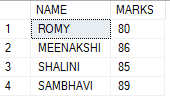Here, 80.9 gets converted to 80, as FLOOR() returns a value less than or equal to the given value but can not return the value greater than the given one.

### By using CAST() function

• CAST(): This function is used to convert the value into a specific data type.

Syntax:

`CAST( value as datatype)`

Query:

`SELECT NAME, CAST(MARKS as INT) AS MARKS FROM geeksforgeeks;`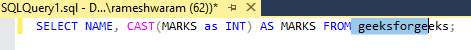Output:This gives results similar to the FLOOR() function. Results vary slightly according to the function used. One should choose according to the need.

My Personal Notes arrow_drop_up